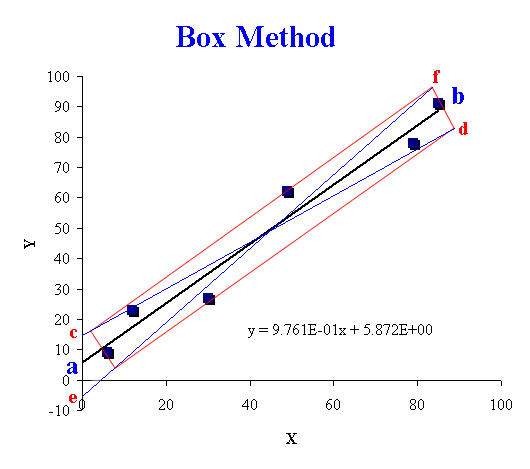Appendix 7: Analysis of error in the slope and the intercept of a linear plot using the 'Box" method.

The 'Box' method is a fast and simple way of determining the uncertainty or error in the slope and intercept of a linear plot. When a plot is made of several points, under experimental conditions in the laboratory, usually there is some amount of scattering. The scattering is usually about some best straight line, drawn so that there is equal (as best as possible) scatter on both sides of it.

NB.The best straight line does not have to touch, or pass through, any of the plotted points.For the above plot, which has six (6) points, the best straight line is shown by the line ab. After the best straight line is drawn, the 'box', which is a rectangle, is drawn so as to just enclose all 6 points. There can only be 1 box that uniquely describes the data and this is chosen using the point lowest from the line and highest above the line and the point to the far left and furthest right as shown.

At this point it would be good to note that if one or two points seem to be very far off the best straight line then it may be possible to 'exclude' them. Tests on significance can be run to determine whether these points, called 'outliers', can be legitamately ignored.

The next step is to find the gradient of the lines forming the diagonals of the 'box'. The line cd is the line of minimum slope and the line ef is the line of maximum slope.

If the slope of the best straight line has a value of S and the error in its value is ΔS, then

Δ S = (max. slope - min. slope) / (2√n)

Similarly, if the value of the intercept is I, and the error in its value is ΔI, then

ΔI = (max. intercept - min. intercept) / (2√n)

n = number of points enclosed in the 'box'.

The fractional error in the slope is given by ΔS/S, and the percentage error in the slope is given by (ΔS/S) x 100. If a parameter P is derived directly from the slope, say, by multiplication or division then ΔS/S = ΔP/P, i.e. the fractional error in the slope has the same value as the fractional error in the parameter P.

Note.
If you are using Microsoft Excel it is possible to obtain these errors using the LINEST function.
1. Enter the X and Y values for which you wish to do the analysis
2. Highlight a range for the output of the analysis (This should be 2 columns wide and 4 rows deep and away from your X,Y points)
3. enter the formula for LINEST.
For example, =LINEST(b2.b12,a2.a12,TRUE,TRUE)
then hit the Control,Shift,Enter keys simultaneously
4. the slope and intercept are in the first row of the output and the error in the slope and the intercept are in the second row of the output. The correlation coefficient is in the third row.
Alternatively, plot the XY data then, after selecting a point, choose Add Trendline from the Chart menu. The first tab shows Type and for this you can select Linear, the second tab shows Options and you can select Display equation on chart and if desired force the intercept through a specific point, e.g. zero.
The output will show only the slope and intercept and not the errors or correlation coefficient.Return to Chemistry, UWI-Mona, Home Page